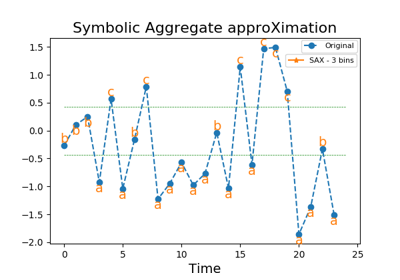# `pyts.approximation`.SymbolicAggregateApproximation¶

class `pyts.approximation.``SymbolicAggregateApproximation`(n_bins=4, strategy='quantile', alphabet=None)[source]

Symbolic Aggregate approXimation.

Parameters: n_bins : int (default = 4) The number of bins to produce. It must be between 2 and `min(n_timestamps, 26)`. strategy : ‘uniform’, ‘quantile’ or ‘normal’ (default = ‘quantile’) Strategy used to define the widths of the bins: ‘uniform’: All bins in each sample have identical widths ‘quantile’: All bins in each sample have the same number of points ‘normal’: Bin edges are quantiles from a standard normal distribution alphabet : None, ‘ordinal’ or array-like, shape = (n_bins,) Alphabet to use. If None, the first n_bins letters of the Latin alphabet are used. If ‘ordinal’, integers are used.

References

 [R2014a3481a12-1] J. Lin, E. Keogh, L. Wei, and S. Lonardi, “Experiencing SAX: a novel symbolic representation of time series”. Data Mining and Knowledge Discovery, 15(2), 107-144 (2007).

Examples

```>>> from pyts.approximation import SymbolicAggregateApproximation
>>> X = [[0, 4, 2, 1, 7, 6, 3, 5],
...      [2, 5, 4, 5, 3, 4, 2, 3]]
>>> transformer = SymbolicAggregateApproximation()
>>> print(transformer.transform(X))
[['a' 'c' 'b' 'a' 'd' 'd' 'b' 'c']
['a' 'd' 'c' 'd' 'b' 'c' 'a' 'b']]
```

Methods

 `__init__`(self[, n_bins, strategy, alphabet]) Initialize self. `fit`(self[, X, y]) Pass. `fit_transform`(self, X[, y]) Fit to data, then transform it. `get_params`(self[, deep]) Get parameters for this estimator. `set_params`(self, \*\*params) Set the parameters of this estimator. `transform`(self, X) Bin the data with the given alphabet.
`__init__`(self, n_bins=4, strategy='quantile', alphabet=None)[source]

Initialize self. See help(type(self)) for accurate signature.

`fit`(self, X=None, y=None)[source]

Pass.

Parameters: X Ignored y Ignored
`fit_transform`(self, X, y=None, **fit_params)

Fit to data, then transform it.

Fits transformer to X and y with optional parameters fit_params and returns a transformed version of X.

Parameters: X : numpy array of shape [n_samples, n_features] Training set. y : numpy array of shape [n_samples] Target values. **fit_params : dict Additional fit parameters. X_new : numpy array of shape [n_samples, n_features_new] Transformed array.
`get_params`(self, deep=True)

Get parameters for this estimator.

Parameters: deep : bool, default=True If True, will return the parameters for this estimator and contained subobjects that are estimators. params : mapping of string to any Parameter names mapped to their values.
`set_params`(self, **params)

Set the parameters of this estimator.

The method works on simple estimators as well as on nested objects (such as pipelines). The latter have parameters of the form `<component>__<parameter>` so that it’s possible to update each component of a nested object.

Parameters: **params : dict Estimator parameters. self : object Estimator instance.
`transform`(self, X)[source]

Bin the data with the given alphabet.

Parameters: X : array-like, shape = (n_samples, n_timestamps) Data to transform. y Ignored X_new : array, shape = (n_samples, n_timestamps) Binned data.

## Examples using `pyts.approximation.SymbolicAggregateApproximation`¶Symbolic Aggregate approXimation